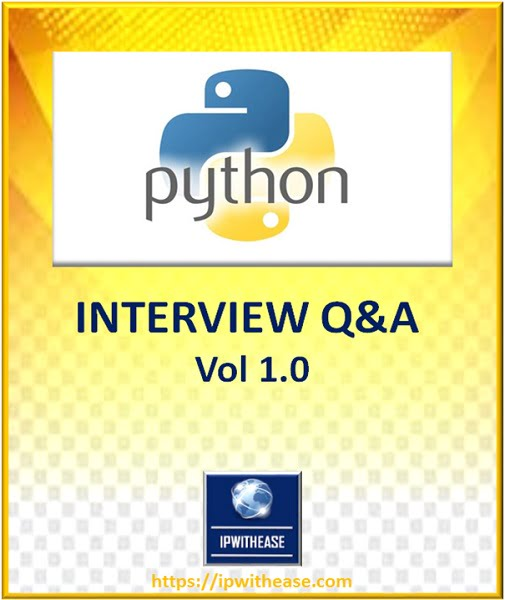# Python Interview Questions

##ANSWERS @https://ipwithease.com/shop/

1. What is python?
2. List some features of python?
3. Is python a case sensitive language?
4. What are the supported built-in data types in Python?
5. What is the output of print str if str = ‘Hello World!’?
6. Write the output of statement print str[2:5] if str = ‘Hello World!’?
7. What will be the output of print str * 2 if str = ‘Hello World!’?
8. What are tuples in Python?
9. List the main differences between tuples and lists in Python?
10. What are Python’s dictionaries?
11. How will you create a dictionary in python?
12. What is the purpose of ** operator?
13. What is the purpose of “is” operator?
14. Which command is used to get a random item from a list or tuple?
15. How will you capitalize first letter of string?
16. Which method is used to remove all leading whitespace in string?
17. Which method is used swap case of all letters in string?
18. How will you reverse a list?
19. What is lambda function in python?
20. Is Python platform independent?
21. Write a function to give the sum of all the numbers in list?

Sample list − (10, 15, 20, 25, 30)

Expected output − 100

22. Write a code in Python to reverse a string without using any built-in function of reverse string?
23. How Python is interpreted?
24. What are some of the tools that help to find bugs or perform static analysis?
25. What is namespace in Python?
26. What is module and package in Python?
27. Explain how can you generate random numbers in Python?
28. Is indentation required in python?
29. What is __init__?
30. What is self in Python?
31. How does break, continue and pass work?
32. What are docstrings in Python?
33. Does Python have OOPs concepts?
34. What are Python libraries? Name a few of them.
35. List the built-in types of python?
36. What is the use of “is”, “not” and “in” operators?
37. Explain Inheritance in Python?
38. How are classes created in Python?
39. What is Polymorphism in Python?
40. Define encapsulation in Python?
41. Explain data abstraction in Python?
42. What is a constructor in OOP?
44. Define exception handling in OOP?
45. What is an Inline function?
46. What is the main difference between a class and an object?
47. What are the access modifiers?
48. What are the various types of constructors?
49. What is ‘this’ pointer?
50. What is static and dynamic Binding?